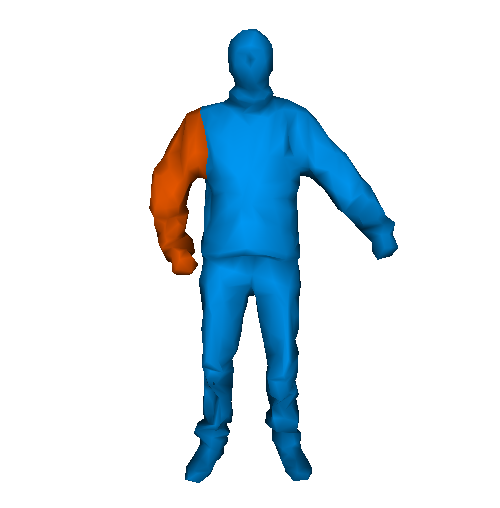EN FR
EN FR

## Section: New Results

### A discrete 3D+t Laplacian framework for mesh animation processing

In this work we extend the discrete 3D Laplacian framework to mesh animations, represented as temporally coherent sequences of meshes (Figure 3 ). In order to let the user control the motion influence with respect to the geometry, we introduce a parameter for the time dimension. Our discrete 3D+t Laplace operator holds the same properties as the discrete 3D Laplacian, as soon as this parameter is non negative. We demonstrate the usefulness of this framework by extending Laplacian-based mesh editing and fairing techniques to mesh animations  .

Figure 3. 3D+t Laplacian